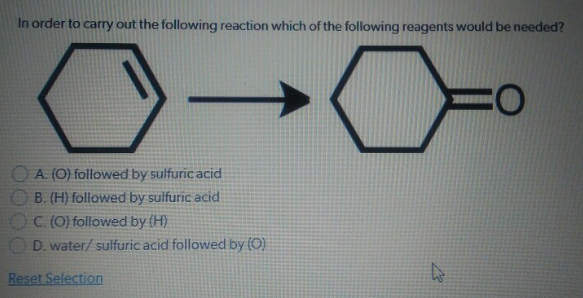# Problem: In order to carry out the following reaction which of the following reagents would be needed? a. (O) followed by sulfuric acid b. (H) followed by sulfuric acid c. (O) followed by (H) d. water/sulfuric acid followed by (O)

###### FREE Expert Solution
83% (145 ratings)###### Problem Details

In order to carry out the following reaction which of the following reagents would be needed?

a. (O) followed by sulfuric acid

b. (H) followed by sulfuric acid

c. (O) followed by (H)

d. water/sulfuric acid followed by (O)